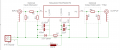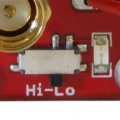# Need help with this circuit please tell me what resistor values for attenuator

Thread Starter

#### born2dive00

Joined Oct 24, 2016
285The RA07M4047M can handle (If I am reading it correctly) from the specs, 50mw of power
https://www.dropbox.com/s/lry7cgj1i8g959b/7wbooster_ra07m4047m.pdf?dl=0

The power coming from the transmitter input is either 200mw low power switched on or 500mw high power switched on.
So as I understand it I need an attenuator that drops the power by 4 or 10 correct?

So for safety sake lets say the input is 500mw what would be the resistor value be???

On the out put side it has a switch to change it from 7watts out put to 3.5wats output, I am guessing this is using the resistors to 1/2 the power out put, so what would be the values on the output side of the attenuator/filter???

How is the switch wired in to the 1/2 the watts?

please let me know Thanks

#### AlbertHall

Joined Jun 4, 2014
11,139

#### LesJones

Joined Jan 8, 2017
3,099
Here is a link to an online calculator for Pi attennuators. As you want a power ratio of 10:1 that is an attennuation of 10 db. The data sheet on the amplifier gives it's input and output impedance as 50 ohms so for that attenuator R1 and R3 would be 96.25 ohms and R2 would be 71.15 ohms. For the power ratio of 4:1 the attennuation would be 6.02 db so R1 & R3 would be 150 ohms and R2 37.5 ohms.
For the output attenuator to give a power of 2:1 the attenuation required would be 3.01 db so R4 & R5 would be 291.5 ohms and R6 17.7 ohms.
At the frequency of 450 Mhz you would have to choose the type of resistor very carefully so it had very low inductance. To switch the attenuator in and out you would need a double pole double throw switch. This would also have to be selected carefully for use at 450 Mhz.

Les.

Thread Starter

#### born2dive00

Joined Oct 24, 2016
285
Here is a link to an online calculator for Pi attennuators. As you want a power ratio of 10:1 that is an attennuation of 10 db. The data sheet on the amplifier gives it's input and output impedance as 50 ohms so for that attenuator R1 and R3 would be 96.25 ohms and R2 would be 71.15 ohms. For the power ratio of 4:1 the attennuation would be 6.02 db so R1 & R3 would be 150 ohms and R2 37.5 ohms.
For the output attenuator to give a power of 2:1 the attenuation required would be 3.01 db so R4 & R5 would be 291.5 ohms and R6 17.7 ohms.
At the frequency of 450 Mhz you would have to choose the type of resistor very carefully so it had very low inductance. To switch the attenuator in and out you would need a double pole double throw switch. This would also have to be selected carefully for use at 450 Mhz.

Les.
Hello Les,
the unit is on 433mhz

I found out that the unit
The output power is configurable by supply voltage and switch. It starts boosting from 3.7v(0.8W) to 9v(10W).
You can supply it with 2S lipo (7.2-8.4v) battery and it gives 6-8W RF depends on battery voltage.

Boosters have selectable gate voltage with a switch
It's including a zener(3.3v) and a blue LED(2.75v) on gain inputwhat type of switch is this? what type of gate is being used? Would standard carbon filter resistors work or would they need to be the micro style? what type or resistor do you recommend? when you speak of very low inductance is this paracitic inductance or resistance inductance?

What is DS10 L1?

Last edited:

#### LesJones

Joined Jan 8, 2017
3,099
If you are no longer using the output attenuator then you will not need to switch it in and out so you don't need a switch suitable for high frequency RF. Any kind of switch will be OK for selecting the supply voltage the the RF amplifier. Carbon composition resistors will probably work. Avoid metal film resistors as some have a helical construction which would increase its inductance. You could Google "resistors for RF" to try to find the most suitable type of resistor. If you do not have experience of building circuits for UHF frequencies then research "UHF construction techniques" DS10 (L1) will be a filter inductor to reduce the amount of RF that finds it way outside of the unit via the power line.

Les.